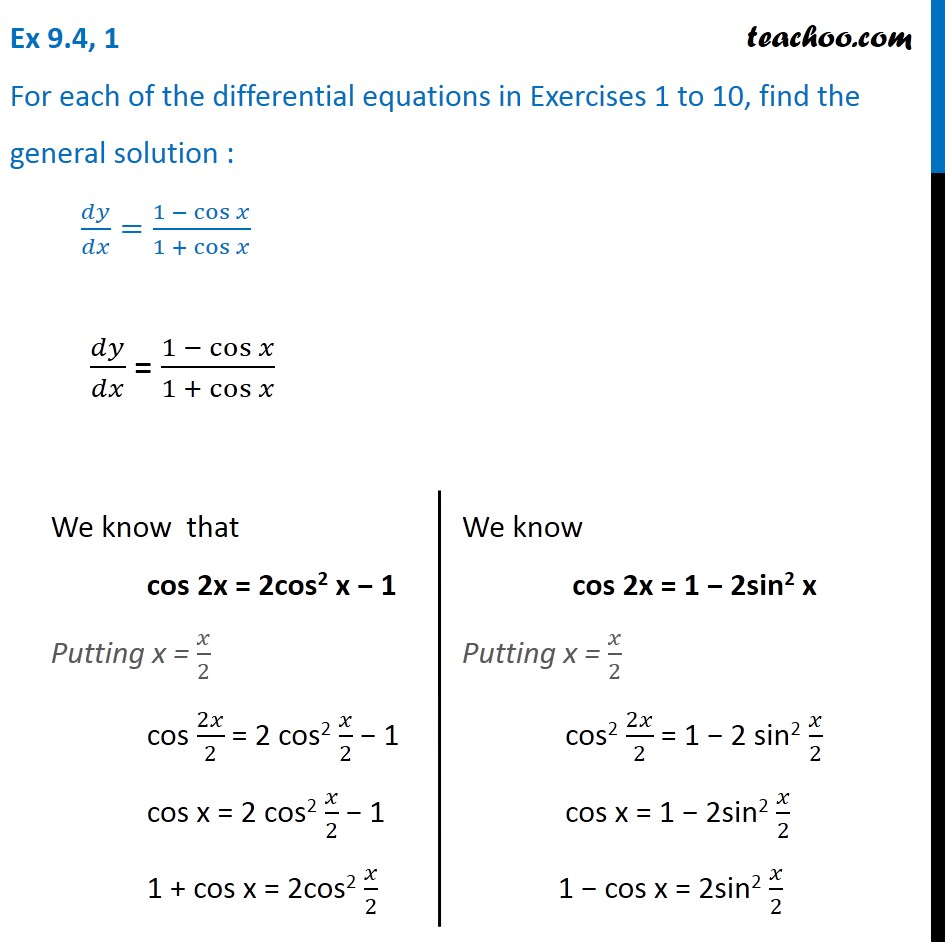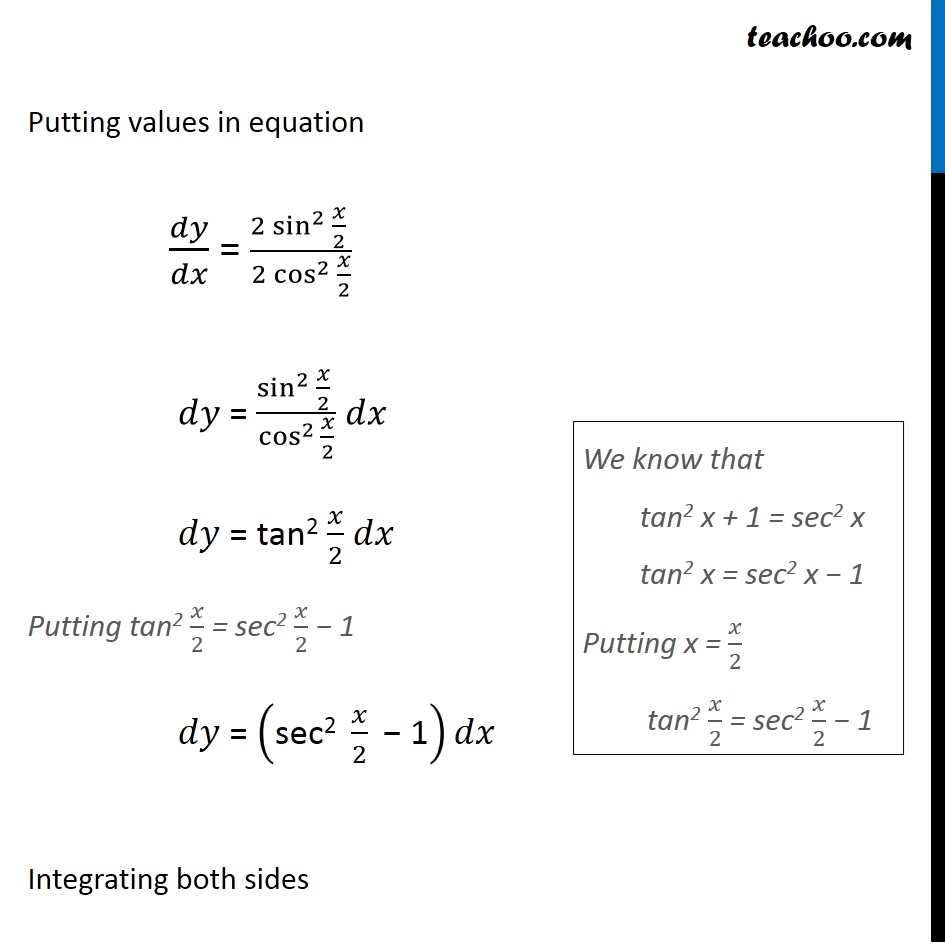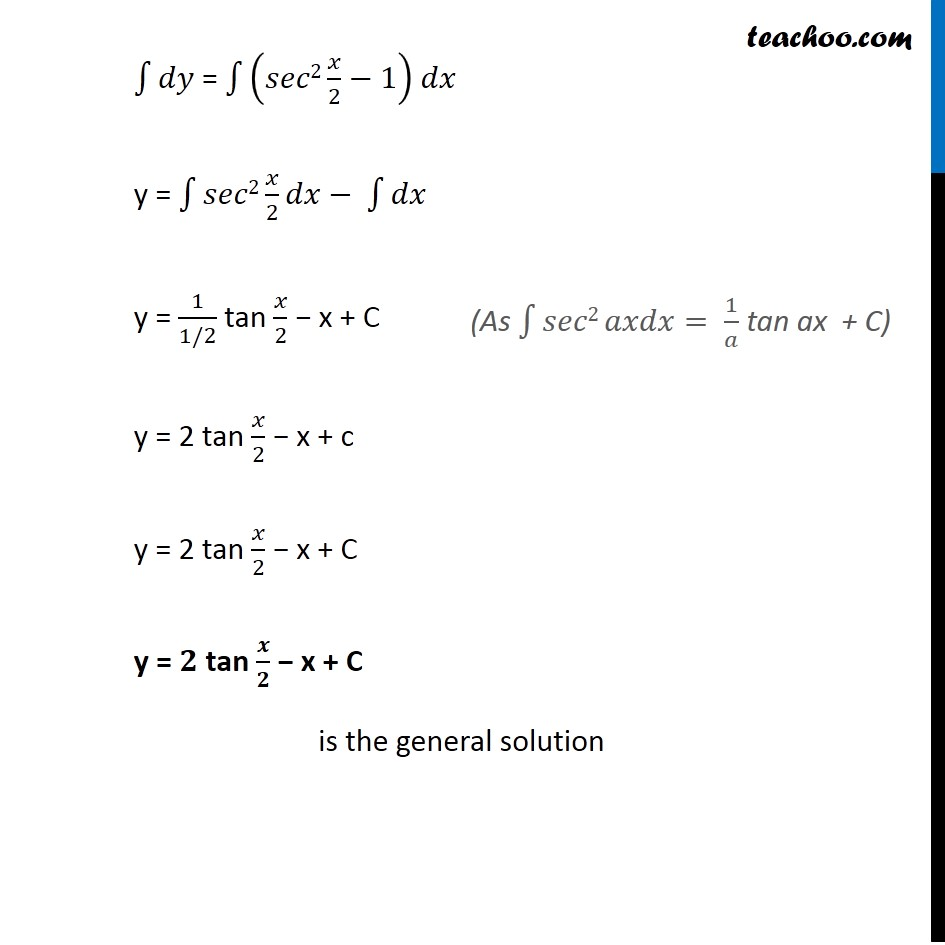1. Chapter 9 Class 12 Differential Equations (Term 2)
2. Serial order wise
3. Ex 9.4

Transcript

Ex 9.4, 1 For each of the differential equations in Exercises 1 to 10, find the general solution : 𝑑𝑦/𝑑𝑥=(1 − cos⁡𝑥)/(1 + cos⁡𝑥 ) 𝑑𝑦/𝑑𝑥 = (1 − cos⁡𝑥)/(1 + cos⁡𝑥 ) We know that cos 2x = 2cos2 x − 1 Putting x = 𝑥/2 cos 2𝑥/2 = 2 cos2 𝑥/2 − 1 cos x = 2 cos2 𝑥/2 − 1 1 + cos x = 2cos2 𝑥/2 We know cos 2x = 1 − 2sin2 x Putting x = 𝑥/2 cos2 2𝑥/2 = 1 − 2 sin2 𝑥/2 cos x = 1 − 2sin2 𝑥/2 1 − cos x = 2sin2 𝑥/2 Putting values in equation 𝑑𝑦/𝑑𝑥 = (2 〖sin^2 〗⁡〖𝑥/2〗 )/(2 〖cos^2 〗⁡〖𝑥/2〗 ) 𝑑𝑦 = (〖sin^2 〗⁡〖𝑥/2〗 )/cos^(2 )⁡〖𝑥/2〗 𝑑𝑥 𝑑𝑦 = tan2 𝑥/2 𝑑𝑥 Putting tan2 𝑥/2 = sec2 𝑥/2 − 1 𝑑𝑦 = ("sec2 " 𝑥/2 " − 1" )𝑑𝑥 Integrating both sides We know that tan2 x + 1 = sec2 x tan2 x = sec2 x − 1 Putting x = 𝑥/2 tan2 𝑥/2 = sec2 𝑥/2 − 1 ∫1▒𝑑𝑦 = ∫1▒(𝑠𝑒𝑐2 𝑥/2−1) 𝑑𝑥 y = ∫1▒〖𝑠𝑒𝑐2 𝑥/2 𝑑𝑥− ∫1▒𝑑𝑥〗 y = 1/(1/2) tan 𝑥/2 − x + C y = 2 tan 𝑥/2 − x + c y = 2 tan 𝑥/2 − x + C y = 𝟐 tan 𝒙/𝟐 − x + C is the general solution (As ∫1▒〖𝑠𝑒𝑐2 𝑎𝑥𝑑𝑥= 1/𝑎〗 tan ax + C)